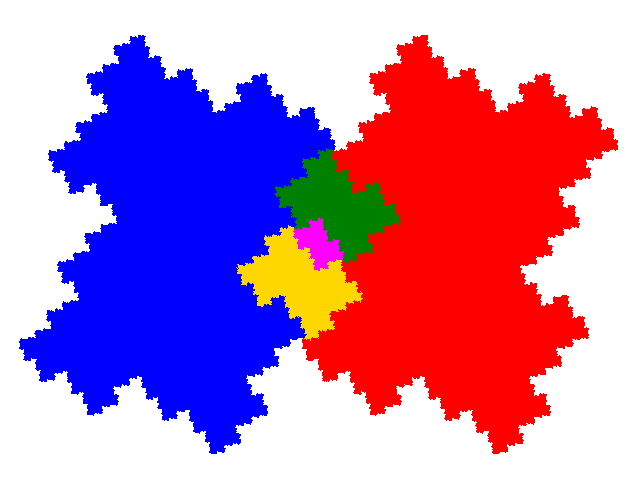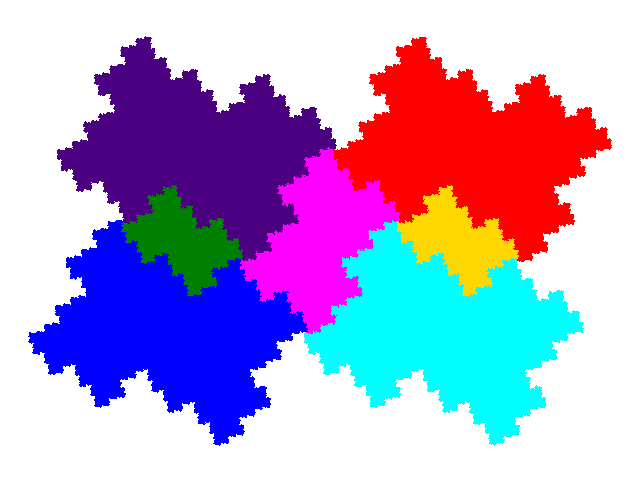# Some tiles associated with the 6th unit cubic Pisot number

## Demisymmetric Tiles (Overview)There are a potential 8 tiles resulting from the application of the grouped element technique to the order 3 symmetric tile, but they come in complementary pairs each of which is the other rotated by 180°, which reduces the number of candidates to 4. Two of these are implementations of the symmetric tile, which leaves us with 2 order 3 demisymmetric tiles.There are a potential 32 tiles resulting from the application of the group element technique to the order 5 symmetric tiles, but this is reduced to 16 by elimination of one copy of each complementary pair, to 12 by elimination of implementations of the symmetric tiles, and to 10 by elimination of order 5 dissections of the order 3 demisymmetric tiles, so there are 10 order 5 demisymmetric tiles.With an order 7 dissection the number of potential tiles rises to 128. This is reduced to 64 (8 groups of 8) by elimination of one copy of each complementary pair, and to 56 by elimination of implementations of the symmetric tile (1 group). In the case of the 2c+2c4+2c7+c9 dissection the remaining 7 groups are all 3 pairs of elements merged (including implementations of 2 order 3 demisymmetric tiles, and 2 order 5 demisymmetric tiles) c1 elements merged c1 and c4 elements merged c1 and c7 elements merged c4 and c7 elements merged c4 elements merged c7 elements merged Thus there are are a total of 52 2c+2c4+2c7+c9 demisymmetric tiles.all three pairs of elements merged (including implementations of 2 order 3 demisymmetric tiles, and 1 disconnected figure) all c2 elements merged (including 3 disconnected figures) long diagonal c2 and c4 elements merged short diagonal c2 and c4 elements merged long diagonal c2 elements merged (including 1 disconnected figure) short diagonal c2 elements merged (including 1 disconnected figure) c4 elements merged Thus there are a total of 48 4c2+c3+2c4 demisymmetric tiles.